Prepare for Any Job Assessment Test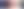# Free Practice Numerical Ability Tests

Updated November 15, 2023

If you are going to sit an aptitude test as part of the job selection process then it will almost certainly include some numerical aptitude questions.

Both the proportion of numerical questions and the type of questions will depend on the job you are applying for.

Obviously, if the job involves dealing with figures on a day-to-day basis then you can expect the proportion of these questions to be quite high.

This will include a wide range of jobs such as those dealing with money, buying, selling, processing invoices, processing orders, administration, engineering, science, statistics and numerical analysis of any sort.

However, even if figure work does not form a substantial part of the job, you will probably still have to answer questions of this type as most employers want some indication of your level of numeracy.

Numerical ability questions are invariably multiple-choice and strictly timed. You will not usually be allowed to use a calculator unless this is expressly stated in the question. These questions can be classified into four types:

##Numerical Computation

These questions test your ability to use the basic principles of arithmetic like addition, subtraction, multiplication and division.

They may also use mathematical terms and methods such as decimals, percentages, ratios, roots, fractions, powers and exponents.

These questions make no attempt to test your reasoning abilities.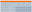Numerical Ability Tests

The method you need to get the correct answer will be obvious and to score well on these questions you will simply need to make quick and accurate calculations.

Numerical Estimation

These questions test your ability to make quick estimates of the answers to fairly straightforward numerical questions.

To score well on these questions you will need to make quick approximations of the answer.

You must avoid the trap of working out the answer exactly, which will take up too much time and prevent you from answering enough questions to get a good score.

If you need to prepare for a number of different employment tests and want to outsmart the competition, choose a Premium Membership from JobTestPrep.

You will get access to three PrepPacks of your choice, from a database that covers all the major test providers and employers and tailored profession packs.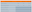Free Practice Numerical Ability Tests

Numerical estimation is key in many craft and technical jobs where the ability to quickly and accurately estimate material quantities is essential.

##Numerical Reasoning

Information is provided that requires you to interpret it and then apply the appropriate logic to answer the questions.

In other words, you need to work out how to get the answer rather than what calculations to apply.

Sometimes the questions are designed to approximate the type of reasoning required in the workplace.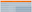Free Practice Numerical Ability Tests

The questions will often use very specific illustrations, for example the question may present financial data or use information technology jargon.

However, an understanding of these areas is not required to answer the question.

Number series questions can also be classified as numerical reasoning questions.

These types of question are very commonly used in graduate and managerial selection.

Data Interpretation

The ability to interpret data presented in tables, graphs and charts is a common requirement in many management and professional jobs.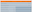Numerical Ability Tests

If you are applying for a job which involves analysis of or decision-making based on numerical data then you can expect to answer this type of question.